﻿ Bending channel with a porous media region (implicit method) - XSim

# Bending channel with a porous media region (implicit method)

OpenFOAM 4.x

$FOAM_TUTORIALS/compressible/rhoPorousSimpleFoam/angledDuctImplicit ## Summary We calculate a flow with a porous material that models a filter in the middle of the flow path. The fluid flows in from the region "inlet" (end face of blue part) at a mass flow rate of 0.1 kg/s, passes through the filter (red part), and flows out from the region "outlet" (end face of green part).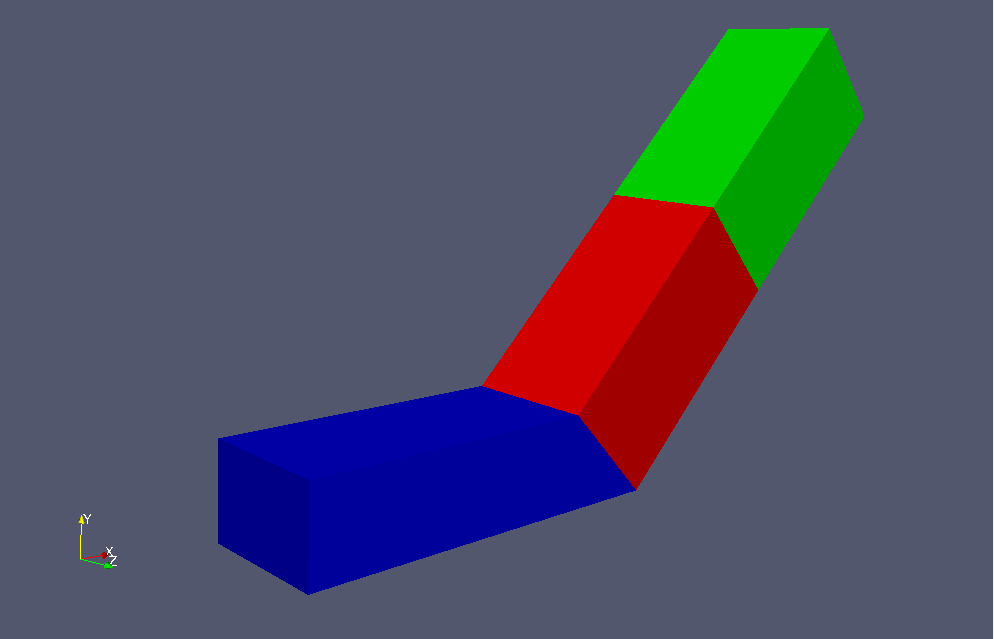Model geometry Porous media depends on the Darcy-Forchheimer law, and for a flow velocity ui (i=x, y, z) in direction i, a generation term Si (pressure drop) in the opposite direction of flow is added to the Navier-Stokes equations. Here, μ is the viscosity coefficient and ρ is the density. The parameters that determine the properties of the porous media, Dij, F, the direction of the properties, and the region in which the porous media, are specified in the file constant/porosityProperties as follows. porosity1 { type DarcyForchheimer; active yes; cellZone porosity; DarcyForchheimerCoeffs { d (5e7 -1000 -1000); f (0 0 0); coordinateSystem { type cartesian; origin (0 0 0); coordinateRotation { type axesRotation; e1 (0.70710678 0.70710678 0); e2 (0 0 1); } } } }  By specifying non-zero for "nUCorrectors" in "SIMPLE" in the file system/fvSolution, the calculation is performed in implicit method instead of explicit method. The standard k-ε model is used for the turbulence model. The meshes are as follows, and the number of mesh is 22000.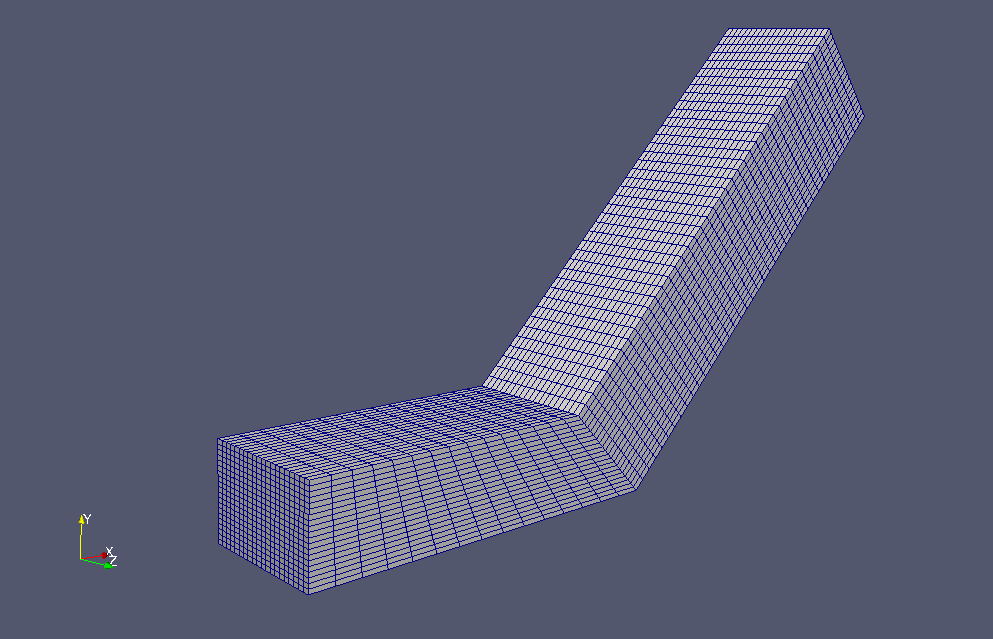Meshes If you want to visualize turbulent energy and turbulent dissipation rate, check "k" and "epsilon" in the "Properties" tab in ParaView.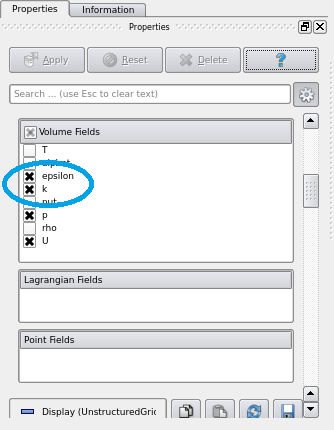Check "k" and "epsilon" in "Properties" tab The calculation result is as follows.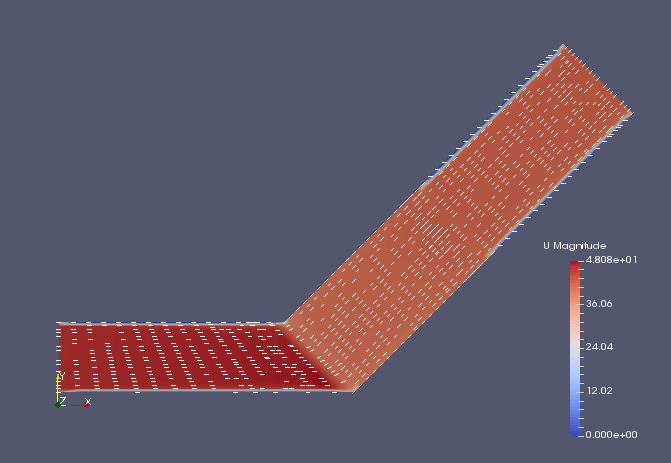Flow velocity (U)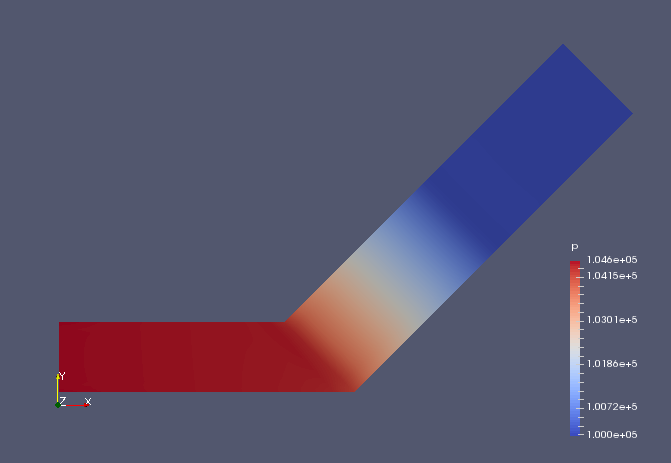Pressure (p)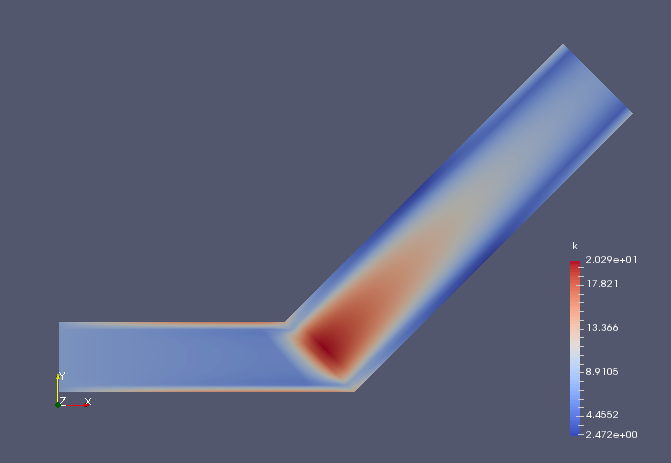Turbulent energy (k)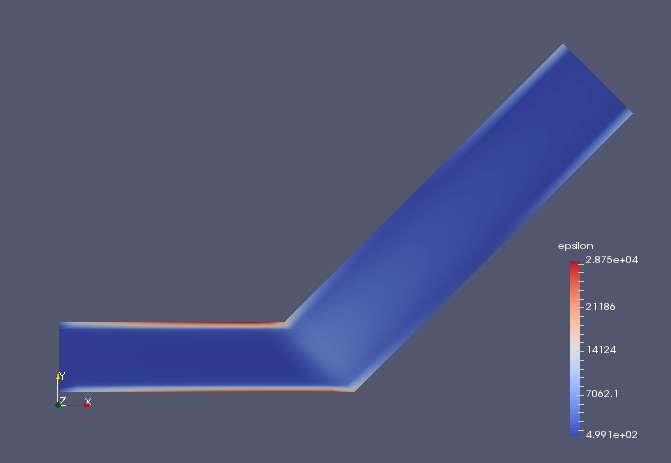Turbulent dissipation rate (epsilon) We can see that the flow velocity and pressure are greatly reduced in the porous media part. ## Commands cp -r$FOAM_TUTORIALS/compressible/rhoPorousSimpleFoam/angledDuctImplicit angledDuctImplicit
cd angledDuctImplicit

m4 system/blockMeshDict.m4 > system/blockMeshDict
blockMesh
rhoPorousSimpleFoam

paraFoam

## Calculation time

2.4 seconds *Single, Inter(R) Core(TM) i7-8700 CPU @ 3.20GHz 3.19GHz